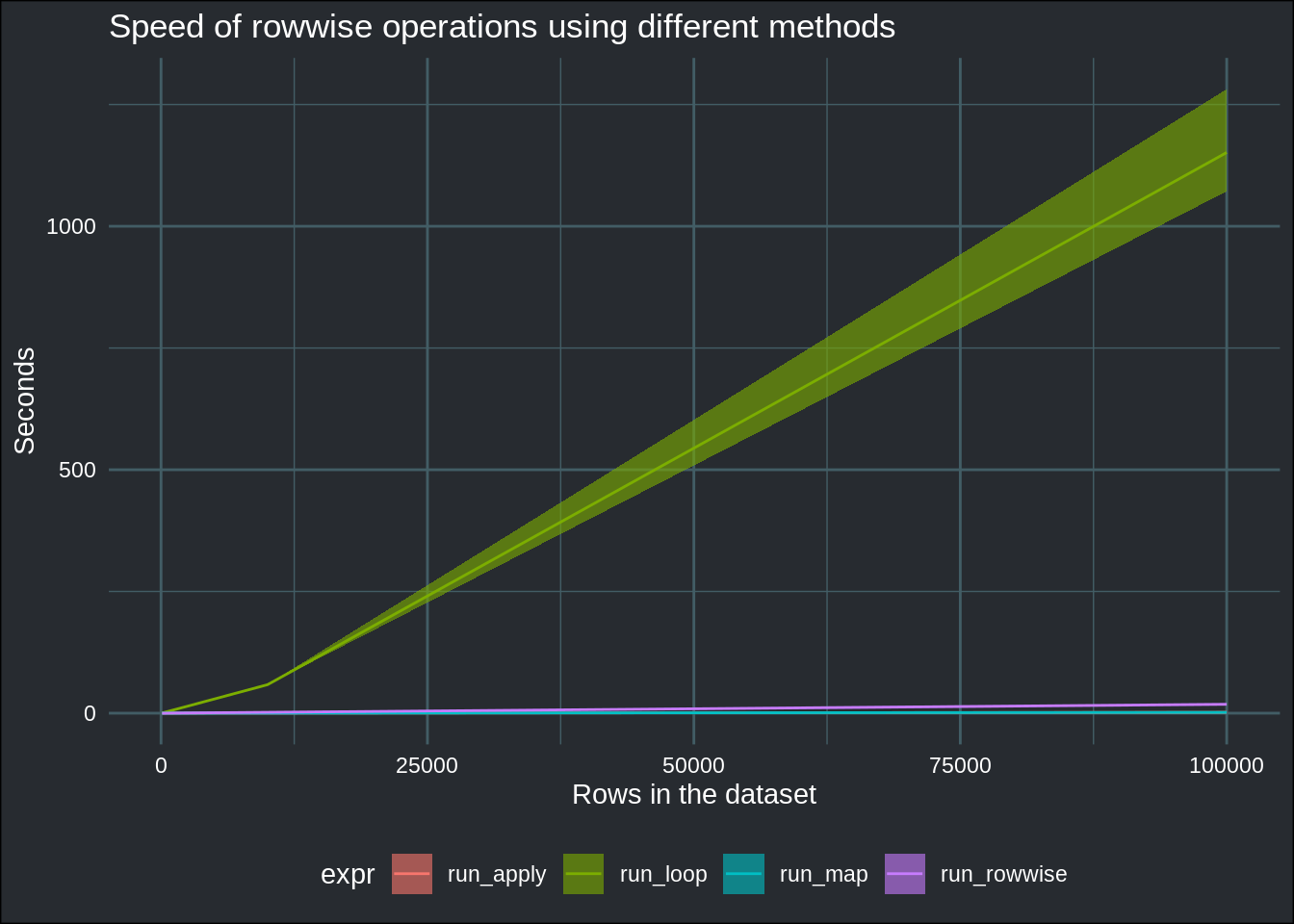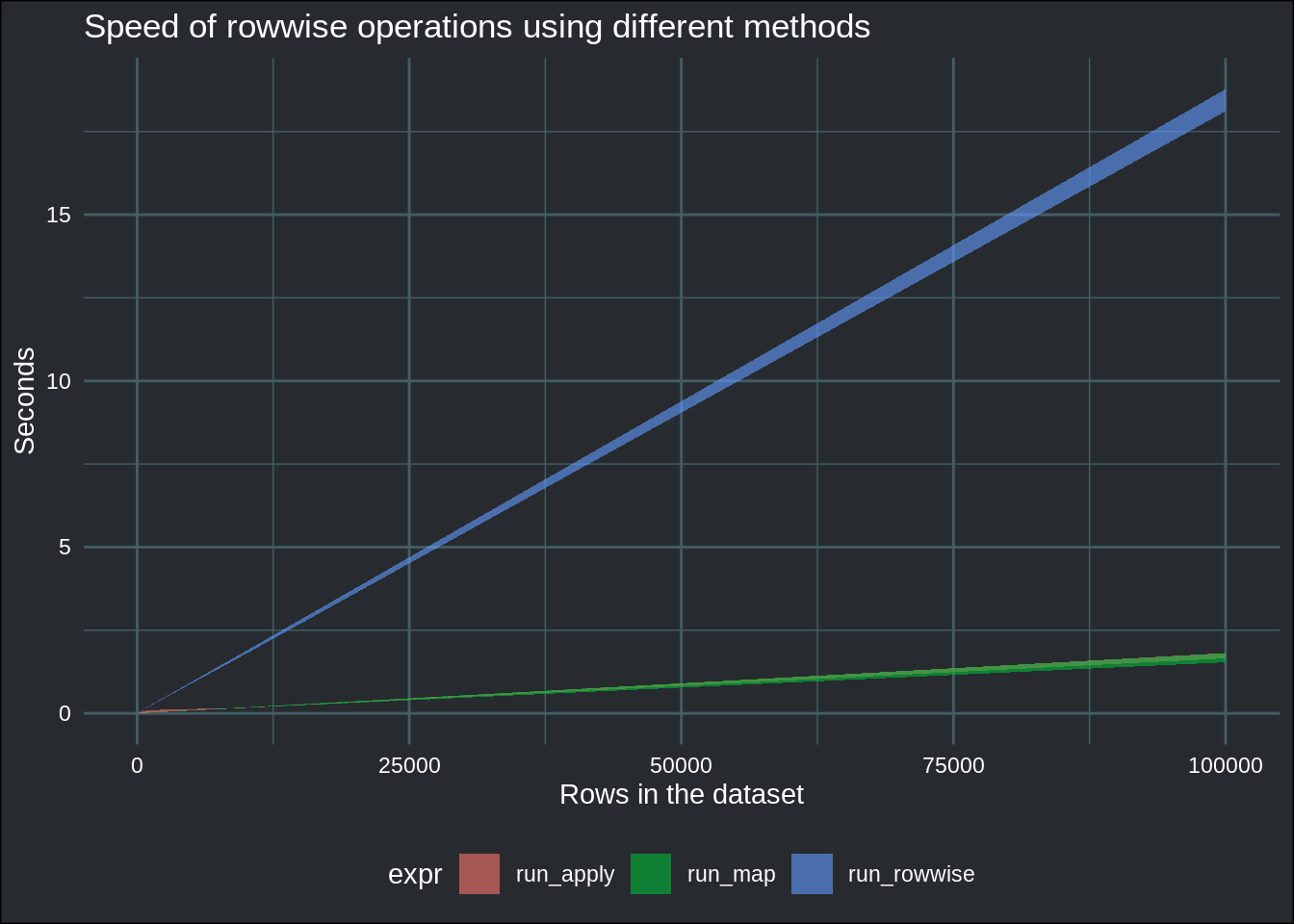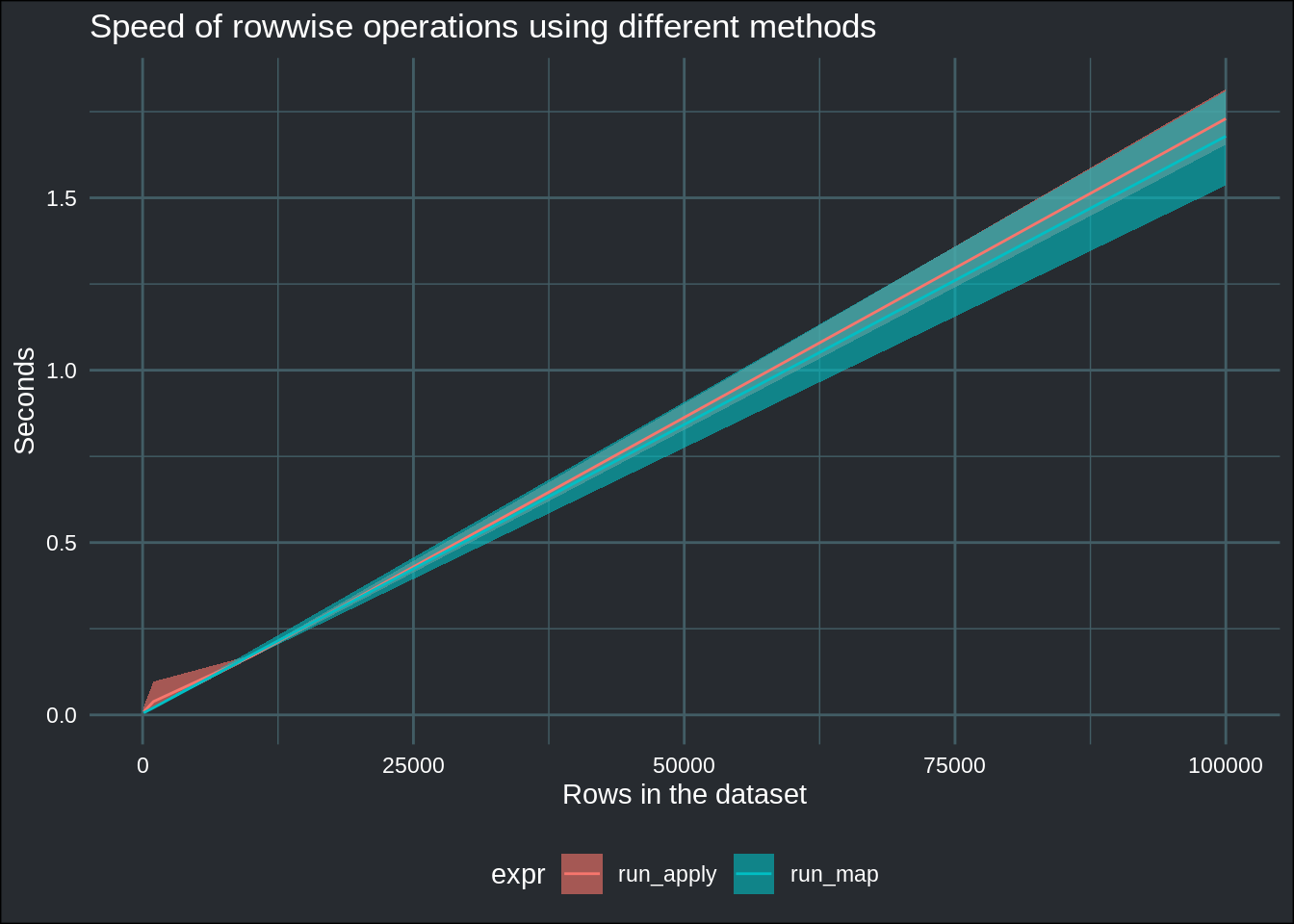```Econometrics and Free Software by Bruno Rodrigues.
Check out my package that adds logging to R functions, {chronicler}.
Or read my free ebooks, to learn some R and build reproducible analytical pipelines..
You can also watch my youtube channel or find the slides to the talks I've given here.
Buy me a coffee, my kids don't let me sleep.
```

# The quest for fast(er?) row-oriented workflows

R

Part 2 of this blog post is available here

The past few weeks I have been exploring the speed of R. It started with this video in which I explained that R is not necessarily slower than any other interpreted language, as long as you’re using the built-in, optimized functions. However should you write your own implementation of an algorithm, especially if that algorithm requires the use of one (or more…) loops, it’ll run slowly. As I’ve also mentioned in two other videos, here and here there are many ways to avoid loops, and you should do so if possible.

To continue exploring this is in more detail, I’ve written two very basic implementations of a genetic algorithm. The first version uses only `{tidyverse}` functions and the other only base R functions. My intuition was that base would be faster, but the code would likely be less “readable” (I discuss this code in greater detail in a series of videos, you can watch part 1 and part 2 if you’re interested in the nitty-gritty details). Code readability is quite subjective, but I think that there are some general “truths” regarding it, namely that it seems to often be that case that fast code is code that is “less” readable, and vice-versa. This blog post explores this trade-off in the context of row-oriented workflows.

Once I was done writing the two versions of the genetic algorithm for the video (a `{tidyverse}` one and a base one), I profiled the code and realised that, yes base was much much faster, but also that the reason the `{tidyverse}` version was running so slowly was because of one single row-based operation. Trying to replace this row-based operation, but remaining inside the `{tidyverse}` made for an interesting challenge. I will explain what I did in this blog post, so first let’s set up the example:

``````library(tidyverse)
library(brotools)``````

Let’s first generate some data. For this, I’m going to use a function I wrote for my genetic algorithm. I won’t explain how it works, so if you’re curious, you can watch the videos I mention in the introduction where this is all explained in detail:

``````init_pop <- function(objective_function, pop_size = 100, upper_bound = 1, lower_bound = 0){

parameters <- formals(objective_function)[] %>%
eval

purrr::rerun(length(parameters), runif(n = pop_size,
min = lower_bound,
max = upper_bound)) %>%
dplyr::bind_cols() %>%
janitor::clean_names()

}``````

This function takes another function, the objective function to be optimized, as an argument, and checks how many parameters this objective functions has, and generates a population of random solutions (if you don’t understand what this all means don’t worry. What matters is that this generates a random dataset whith as many columns as the objective function has arguments).

The next function is my objective function:

``````my_function <- function(x = c(0, 0, 0, 0, 0, 0)){
x1 <- x
x2 <- x
x3 <- x
x4 <- x
x5 <- x
x6 <- x

-(x1**2 + x2 - 11)**2 - (x1 + x2**2 - 7)**2 - (x3**3 + x4 - 11)**2 - (x5 + x6**2 - 7)**2
}``````

(This is not the same as in the videos, which only has two arguments.)

Let’s generate some data:

``````dataset <- init_pop(my_function) %>%
as.data.frame()``````
``head(dataset)``
``````##          x1        x2          x3        x4         x5        x6
## 1 0.3045714 0.1436057 0.003754794 0.9144551 0.53070392 0.6127125
## 2 0.3155244 0.8890011 0.556325257 0.5688512 0.02928638 0.5626903
## 3 0.8363487 0.6361570 0.667718047 0.4704217 0.10547741 0.5278469
## 4 0.8207208 0.1286540 0.189744816 0.3309174 0.76311349 0.7019268
## 5 0.7244419 0.1284358 0.235967085 0.8444759 0.38697023 0.9818212
## 6 0.2882561 0.9702481 0.983408531 0.1510577 0.84844059 0.7678110``````

Now, on the actual problem: I need to add another column, with the value of `my_function()`, evaluated on a per row basis. As an example, for the first row, this would be the result of:

``my_function(dataset[1, ])``
``````##          x1
## 1 -299.2624``````

Many people would probably solve this using a for loop, so let’s write a function to do just that (benchmarking will make it easier if the code is inside a function):

``````run_loop <- function(dataset, my_function = my_function){

dataset\$score <- 0

for(i in seq(1, nrow(dataset))){

dataset\$score[i] <- my_function(dataset[i, ])
}

dataset

}

run_loop(dataset, my_function = my_function) %>%
``````##          x1        x2          x3        x4         x5        x6     score
## 1 0.3045714 0.1436057 0.003754794 0.9144551 0.53070392 0.6127125 -299.2624
## 2 0.3155244 0.8890011 0.556325257 0.5688512 0.02928638 0.5626903 -284.4934
## 3 0.8363487 0.6361570 0.667718047 0.4704217 0.10547741 0.5278469  -275.027
## 4 0.8207208 0.1286540 0.189744816 0.3309174 0.76311349 0.7019268 -288.6529
## 5 0.7244419 0.1284358 0.235967085 0.8444759 0.38697023 0.9818212 -281.0109
## 6 0.2882561 0.9702481 0.983408531 0.1510577 0.84844059 0.7678110 -261.1376``````

The advantage of loops is that you don’t need to really know a lot about R to get it done; if you’ve learned some programming language some time during your studies, you learned about for loops. But they’re generally slower than other methods and error-prone (typos for example, or if you’re looping over several indeces, it can get quite complex…). And they’re, in my humble opinion, not always very easy to understand. This is not the case here, because it is quite a simple example, but often, it can get quite confusing to understand what is going on.

So what would be a more “R-specific” way of doing it (specific in the sense that it is not a universal solution like a for-loop), and which avoids using a loop? `apply()` would here be the best candidate:

``apply(dataset, MARGIN = 1, FUN = my_function)``
``````##    -299.2624 -284.4934 -275.0270 -288.6529 -281.0109 -261.1376 -293.7069
##    -264.7833 -270.6977 -258.5214 -299.6117 -275.8491 -306.8555 -284.7410
##   -298.6167 -299.2872 -294.9865 -264.5808 -272.8924 -289.5542 -306.3602
##   -293.4290 -305.9189 -276.9193 -286.1938 -291.7530 -289.3610 -290.8470
##   -303.5995 -261.4664 -280.6596 -287.2716 -282.6859 -293.5323 -304.2287
##   -286.9913 -258.3523 -275.9231 -304.3919 -250.9952 -286.7151 -255.0904
##   -312.2109 -254.5034 -255.9284 -287.8201 -285.9853 -290.8199 -309.0086
##   -311.4288 -271.1889 -299.3821 -290.1711 -281.0423 -294.1406 -275.8203
##   -274.1912 -257.7994 -308.3508 -271.5294 -293.3045 -296.9122 -277.8800
##   -296.9870 -314.1470 -270.0065 -288.3262 -252.3774 -263.9164 -286.9263
##   -302.5980 -281.0731 -269.0754 -301.6335 -294.3153 -268.4932 -263.6926
##   -306.9723 -271.8796 -292.6175 -294.0995 -303.4289 -280.5853 -277.6487
##   -262.2476 -310.0217 -281.7774 -292.7697 -295.8509 -269.0880 -253.2403
##   -279.8632 -293.0479 -258.1470 -303.6226 -306.4314 -293.4026 -275.8508
##   -269.6470 -285.0784``````

Appending this to a dataframe can be done within a `mutate()` call (here again I’m encapsulating this inside a function, for benchmarking purposes):

``````run_apply <- function(dataset, my_function = my_function){

dataset %>%
mutate(score = apply(., MARGIN = 1, my_function))

}

run_apply(dataset, my_function = my_function) %>%
``````##          x1        x2          x3        x4         x5        x6     score
## 1 0.3045714 0.1436057 0.003754794 0.9144551 0.53070392 0.6127125 -299.2624
## 2 0.3155244 0.8890011 0.556325257 0.5688512 0.02928638 0.5626903 -284.4934
## 3 0.8363487 0.6361570 0.667718047 0.4704217 0.10547741 0.5278469 -275.0270
## 4 0.8207208 0.1286540 0.189744816 0.3309174 0.76311349 0.7019268 -288.6529
## 5 0.7244419 0.1284358 0.235967085 0.8444759 0.38697023 0.9818212 -281.0109
## 6 0.2882561 0.9702481 0.983408531 0.1510577 0.84844059 0.7678110 -261.1376``````

`MARGIN = 1` means that the function is applied on the rows, whereas `MARGIN = 2` would apply the function over columns.

In terms of readability, I think that this is maybe a bit less readable than the for-loop, just because for-loops as very very ubiquitous. But it’s super simple once you understand how `apply()` works.

Now, what would be a `{tidyverse}`-only approach? And why would you want to do a `{tidyverse}`-only approach anyways? Generally, I would argue that scripts written using `{tidyverse}` functions and style are easier to read. For example, I tweeted this code snippet some time ago:

``````blogdown::shortcode("tweet",
"1431718740341764099"
)``````

and in my opinion the example in my tweet shows clearly that the `{tidyverse}` code is more easily understood and readable. Of course, some people disagree… However, in this case here, I’m not sure that a `{tidyverse}` approach would be more readable. The solution using `apply()` seems to me to be quite good. Let’s see how the `{tidyverse}` approach, which leverages `rowwise()`, looks like:

``````run_rowwise <- function(dataset, my_function = my_function){
dataset %>%
rowwise() %>%
mutate(score = my_function(c_across(everything()))) %>%
ungroup()
}

run_rowwise(dataset, my_function = my_function) %>%
``````## # A tibble: 6 × 7
##      x1    x2      x3    x4     x5    x6 score
##   <dbl> <dbl>   <dbl> <dbl>  <dbl> <dbl> <dbl>
## 1 0.305 0.144 0.00375 0.914 0.531  0.613 -299.
## 2 0.316 0.889 0.556   0.569 0.0293 0.563 -284.
## 3 0.836 0.636 0.668   0.470 0.105  0.528 -275.
## 4 0.821 0.129 0.190   0.331 0.763  0.702 -289.
## 5 0.724 0.128 0.236   0.844 0.387  0.982 -281.
## 6 0.288 0.970 0.983   0.151 0.848  0.768 -261.``````

This runs, but much, much, more slower than with `apply()` (but faster than a for-loop, as we shall see) . Plus, it does look much, much more complicated than the simple `apply()` version! So why do it like this? You even need several functions - `rowwise()`, `c_across()` and `everything()` - to make it work! So why? Well, there is one use case in which this approach enables you to do something that I don’t think is possible (or at least easily possible) with `apply()` which is applying the function, but only over certain columns. For example, if you want to apply the function only over the columns which names all start with the letter “c”, you could write something like this:

``````mtcars %>%
rowwise() %>%
mutate(score = mean(c_across(starts_with("c")))) %>%
ungroup()``````
``````## # A tibble: 32 × 12
##      mpg   cyl  disp    hp  drat    wt  qsec    vs    am  gear  carb score
##    <dbl> <dbl> <dbl> <dbl> <dbl> <dbl> <dbl> <dbl> <dbl> <dbl> <dbl> <dbl>
##  1  21       6  160    110  3.9   2.62  16.5     0     1     4     4   5
##  2  21       6  160    110  3.9   2.88  17.0     0     1     4     4   5
##  3  22.8     4  108     93  3.85  2.32  18.6     1     1     4     1   2.5
##  4  21.4     6  258    110  3.08  3.22  19.4     1     0     3     1   3.5
##  5  18.7     8  360    175  3.15  3.44  17.0     0     0     3     2   5
##  6  18.1     6  225    105  2.76  3.46  20.2     1     0     3     1   3.5
##  7  14.3     8  360    245  3.21  3.57  15.8     0     0     3     4   6
##  8  24.4     4  147.    62  3.69  3.19  20       1     0     4     2   3
##  9  22.8     4  141.    95  3.92  3.15  22.9     1     0     4     2   3
## 10  19.2     6  168.   123  3.92  3.44  18.3     1     0     4     4   5
## # … with 22 more rows``````

Now this is not needed here, so `apply()` clearly wins in terms readability (and speed as well). But in cases like the above, where you need to compute only over several columns, I think that the `{tidyverse}` version not only is very readible, but actually offers a solution to the problem. I’m not quite sure you could solve this easily with base, but please prove me wrong.

In any case, there’s another way to approach our original problem using `{tidyverse}` functions, but we still need the help of a base function.

The next approach uses the fact that `map()` needs both a list and a function as an input. As a refresher, here’s how map works:

``````# We have a list

my_list <- list("a" = 2,
"b" = 4)

# and we have a function, say sqrt, which we want to apply to each element of this list

map(my_list, sqrt)``````
``````## \$a
##  1.414214
##
## \$b
##  2``````

So what we need is a way to mimick the basic approach which works on one “element” (in this case, a row of the dataframe), and extend that idea to a “list of rows”. Now, the issue is that a dataframe is actually a list of columns, not rows. So if you’re using `map()` over a dataframe, you will be looping over the columns, not the rows, as in the example below:

``````# This applies the function class() to each colum of mtcars
mtcars %>%
map(class)``````
``````## \$mpg
##  "numeric"
##
## \$cyl
##  "numeric"
##
## \$disp
##  "numeric"
##
## \$hp
##  "numeric"
##
## \$drat
##  "numeric"
##
## \$wt
##  "numeric"
##
## \$qsec
##  "numeric"
##
## \$vs
##  "numeric"
##
## \$am
##  "numeric"
##
## \$gear
##  "numeric"
##
## \$carb
##  "numeric"``````

Now the question becomes; is there a way to turn a dataframe, which is a list of columns, into a list of rows? Yes, there is, using `asplit()`:

``````asplit(mtcars, MARGIN = 1) %>%
``````## \$`Mazda RX4`
##    mpg    cyl   disp     hp   drat     wt   qsec     vs     am   gear   carb
##  21.00   6.00 160.00 110.00   3.90   2.62  16.46   0.00   1.00   4.00   4.00
##
## \$`Mazda RX4 Wag`
##     mpg     cyl    disp      hp    drat      wt    qsec      vs      am    gear
##  21.000   6.000 160.000 110.000   3.900   2.875  17.020   0.000   1.000   4.000
##    carb
##   4.000
##
## \$`Datsun 710`
##    mpg    cyl   disp     hp   drat     wt   qsec     vs     am   gear   carb
##  22.80   4.00 108.00  93.00   3.85   2.32  18.61   1.00   1.00   4.00   1.00
##
## \$`Hornet 4 Drive`
##     mpg     cyl    disp      hp    drat      wt    qsec      vs      am    gear
##  21.400   6.000 258.000 110.000   3.080   3.215  19.440   1.000   0.000   3.000
##    carb
##   1.000
##
##    mpg    cyl   disp     hp   drat     wt   qsec     vs     am   gear   carb
##  18.70   8.00 360.00 175.00   3.15   3.44  17.02   0.00   0.00   3.00   2.00
##
## \$Valiant
##    mpg    cyl   disp     hp   drat     wt   qsec     vs     am   gear   carb
##  18.10   6.00 225.00 105.00   2.76   3.46  20.22   1.00   0.00   3.00   1.00``````

`asplit()` splits a dataframe along rows (with the MARGIN argument set to 1) or along columns (with MARGIN = 2). As you can see with the code above, the `mtcars` dataset is now a list of rows. Each element of this list is a single vector of values. Now that my dataframe is now a list of rows, well, I can simply use `map()` to apply any function over its rows:

``````run_map <- function(dataset, my_function = my_function){
dataset %>%
mutate(score = map_dbl(asplit(., 1), .f = my_function))
}

run_map(dataset, my_function = my_function) %>%
``````##          x1        x2          x3        x4         x5        x6     score
## 1 0.3045714 0.1436057 0.003754794 0.9144551 0.53070392 0.6127125 -299.2624
## 2 0.3155244 0.8890011 0.556325257 0.5688512 0.02928638 0.5626903 -284.4934
## 3 0.8363487 0.6361570 0.667718047 0.4704217 0.10547741 0.5278469 -275.0270
## 4 0.8207208 0.1286540 0.189744816 0.3309174 0.76311349 0.7019268 -288.6529
## 5 0.7244419 0.1284358 0.235967085 0.8444759 0.38697023 0.9818212 -281.0109
## 6 0.2882561 0.9702481 0.983408531 0.1510577 0.84844059 0.7678110 -261.1376``````

So we now have 4 approaches to solve the issue:

• `run_loop()`: uses a for-loop
• `run_apply()`: uses `apply()`, a base R function
• `run_rowwise()`: a “pure” `{tidyverse}` approach
• `run_map()`: a cross between a `{tidyverse}` and a base approach

Let’s set up a function to run some benchmarks and see which runs faster. I’ll create a list of increasingly large data frames over which I’ll run all the above functions:

``````list_datasets <- map(seq(2, 5), ~init_pop(objective_function = my_function,
pop_size = `^`(10, .x)))``````

The function below will run the benchmarks over all the data frames:

``````run_benchmarks <- function(dataset, times = 5){
microbenchmark::microbenchmark(
run_loop(dataset, my_function = my_function),
run_apply(dataset, my_function = my_function),
run_rowwise(dataset, my_function = my_function),
run_map(dataset, my_function = my_function),
times = times,
unit = "s"
)
}``````

I’ll run this in parallel using `{furrr}`:

``````library(furrr)

plan(multisession, workers = 2)

benchmark_results <- future_map(list_datasets, run_benchmarks)``````

Let’s take a look at the results:

``````benchmark_data <- map2(.x = benchmark_results, .y = 10^seq(2, 5), .f = ~mutate(tibble(.x), pop_size = .y)) %>%
bind_rows() %>%
mutate(expr = str_remove_all(expr, "\\(.*\\)")) %>%
group_by(expr, pop_size) %>%
mutate(time_seconds = time/10^9) %>%
summarise(fastest_run = min(time_seconds),
average_run = mean(time_seconds),
slowest_run = max(time_seconds))``````
``## `summarise()` has grouped output by 'expr'. You can override using the `.groups` argument.``
``````benchmark_data %>%
ggplot(aes(y = average_run, x = pop_size)) +
geom_ribbon(aes(ymin = fastest_run, ymax = slowest_run, fill = expr), alpha = .6) +
geom_line(aes(group = expr, col = expr)) +
ylab("Seconds") +
xlab("Rows in the dataset") +
ggtitle("Speed of rowwise operations using different methods") +
theme_blog()``````Using a for-loop for row-wise operations is clearly the slowest solution. Let’s take a closer look at the remaining 3 options:

``````benchmark_data %>%
filter(!grepl("loop", expr)) %>%
ggplot(aes(y = average_run, x = pop_size)) +
geom_ribbon(aes(ymin = fastest_run, ymax = slowest_run, fill = expr), alpha = .6) +
ylab("Seconds") +
xlab("Rows in the dataset") +
ggtitle("Speed of rowwise operations using different methods") +
theme_blog()```````rowwise()` loses here, but unless you have to literally run such code hundreds of times, it is still tolerable. Gives you enough time to browse some memes. But if you have to run such operations millions of times, you might want to look at either using `apply()` or the other approach that uses `asplit()` and `map()`. Let’s take a closer look at these two:

``````benchmark_data %>%
filter(!grepl("loop|rowwise", expr)) %>%
ggplot(aes(y = average_run, x = pop_size)) +
geom_ribbon(aes(ymin = fastest_run, ymax = slowest_run, fill = expr), alpha = .6) +
geom_line(aes(group = expr, col = expr)) +
ylab("Seconds") +
xlab("Rows in the dataset") +
ggtitle("Speed of rowwise operations using different methods") +
theme_blog()``````Interestingly, the fastest run using `map()` was faster than the fastest run using `apply()`, but on average, both methods seem to be equivalent. In conclusion, if you need speed and you need to compute over every column `apply()` is a clear winner. But if you need row-wise operations, but only on a subset of columns, `rowwise()`, even though it is slow, seems to be the only solution.

I wonder if there is a way to use `c_across()` with the `map()` approach, and potentially have the benefits of `map()` (as fast as `apply()`) and `rowwise()` (computing only over certain columns…). Another subject to explore later.

Part 2 of this blog post is available here

Hope you enjoyed! If you found this blog post useful, you might want to follow me on twitter for blog post updates and buy me an espresso or paypal.me, or buy my ebook on Leanpub. You can also watch my videos on youtube. So much content for you to consoom!